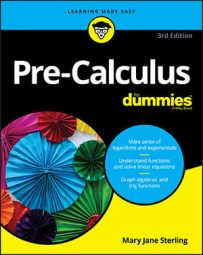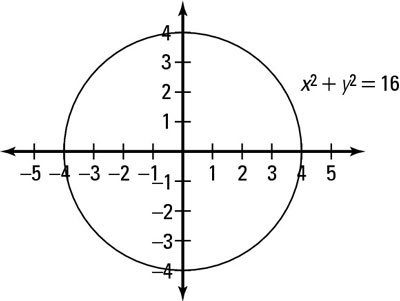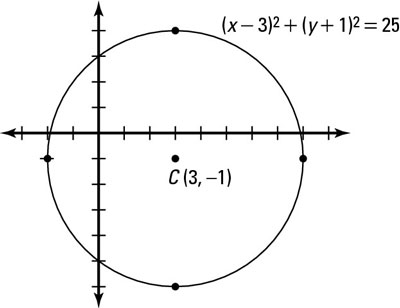##### Pre-Calculus For DummiesCircles are simple to work with in pre-calculus. A circle has one center, one radius, and a whole lot of points, but you follow slightly different steps, depending on whether you are graphing a circle centered at the origin or away from the origin.

The first thing you need to know in order to graph the equation of a circle is where on a plane the center is located. The equation of a circle appears as (x – h)2 + (y – v)2 = r2. This is called the center-radius form (or standard form) because it gives you both pieces of information at the same time.

The h and v represent the coordinates of the center of the circle being at the point (h, v), and r represents the radius. Specifically, h represents the horizontal displacement — how far to the left or to the right of the y-axis the center of the circle is. The variable v represents the vertical displacement — how far above or below the x-axis the center falls.

From the center, you can count from the center r units (the radius) horizontally in both directions and vertically in both directions to get four different points, all equidistant from the center. Connect these four points with the best curve that you can sketch to get the graph of the circle.

## Graphing circles centered at the origin

The simplest circle to graph is one whose center is at the origin (0, 0). Because both h and v are zero, they can disappear and you can simplify the standard circle equation to look like x2 + y2 = r2. For instance, to graph the circle x2 + y2 = 16, follow these steps:
1. Realize that the circle is centered at the origin (no h and v) and place this point there.

2. Calculate the radius by solving for r.

Set r2 = 16. In this case, you get r = 4.

3. Plot the radius points on the coordinate plane.

You count out 4 in every direction from the center (0, 0): left, right, up, and down.

4. Connect the dots to graph the circle using a smooth, round curve.Graphing a circle centered at the origin

The figure shows this circle on the plane.

## Graphing circles centered away from the origin

Although graphing circles at the origin is easiest, very few graphs are as straightforward and simple as those. In pre-calculus, you work with transforming graphs of all different shapes and sizes. Fortunately, these graphs all follow the same pattern for horizontal and vertical shifts, so you don't have to remember many rules.

Don't forget that the coordinates of the center of the circle are of the opposite signs of the h and v from inside the parentheses in the equation. Because the h and v are inside the grouping symbols, this means that the shift happens opposite from what you would think.

For example, follow these steps to graph the equation (x – 3)2 + (y + 1)2 = 25:
1. Locate the center of the circle from the equation (h, v).

(x – 3)2 means that the x-coordinate of the center is positive 3.

(y + 1)2 means that the y-coordinate of the center is negative 1.

Place the center of the circle at (3, –1).

2. Calculate the radius by solving for r.

Set r2 = 25 and square root both sides to get r = 5.

3. Plot the radius points on the coordinate plane.

Count 5 units up, down, left, and right from the center at (3, –1). This step gives you points at (8, –1), (–2, –1), (3, –6), and (3, 4).

4. Connect the dots to the graph of the circle with a round, smooth curve.Graphing a circle not centered at the origin

The figure shows a visual representation of this circle.